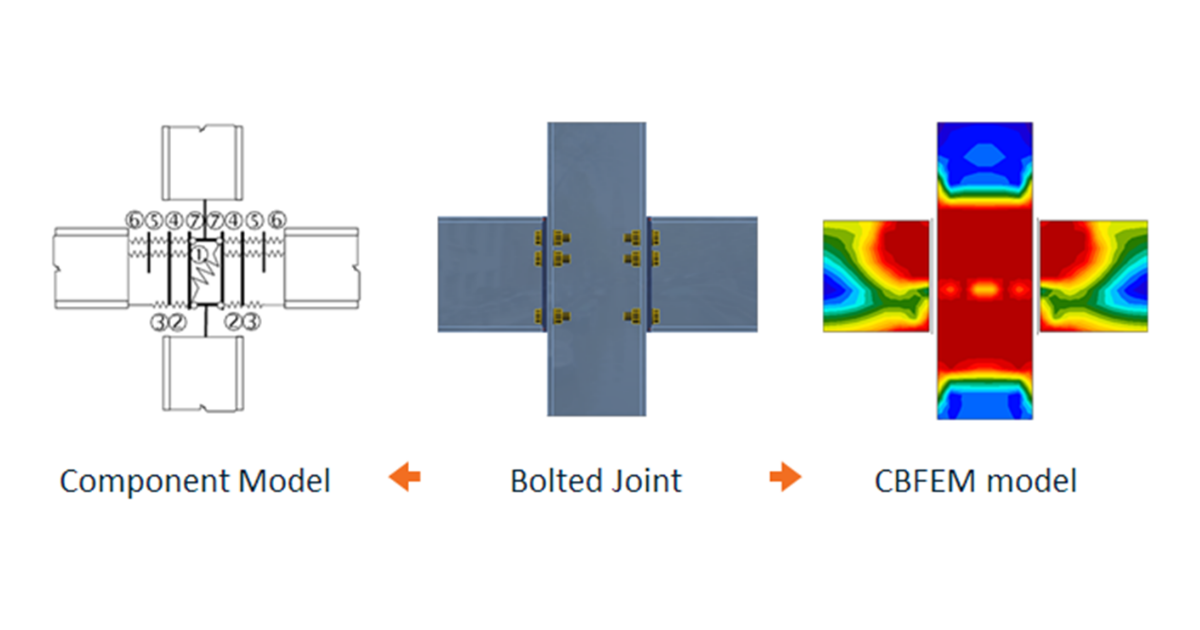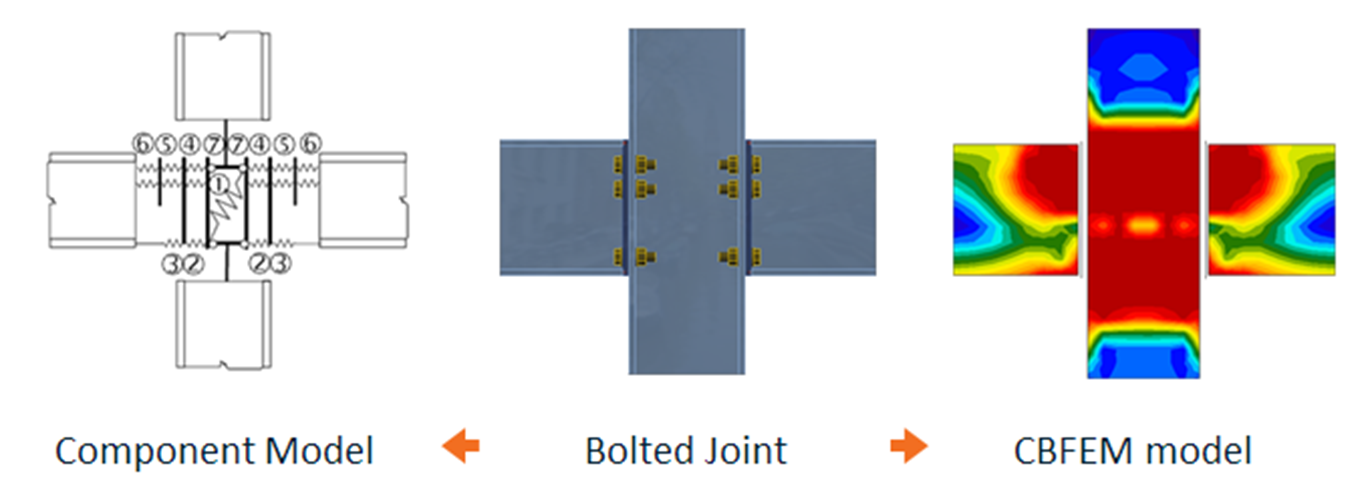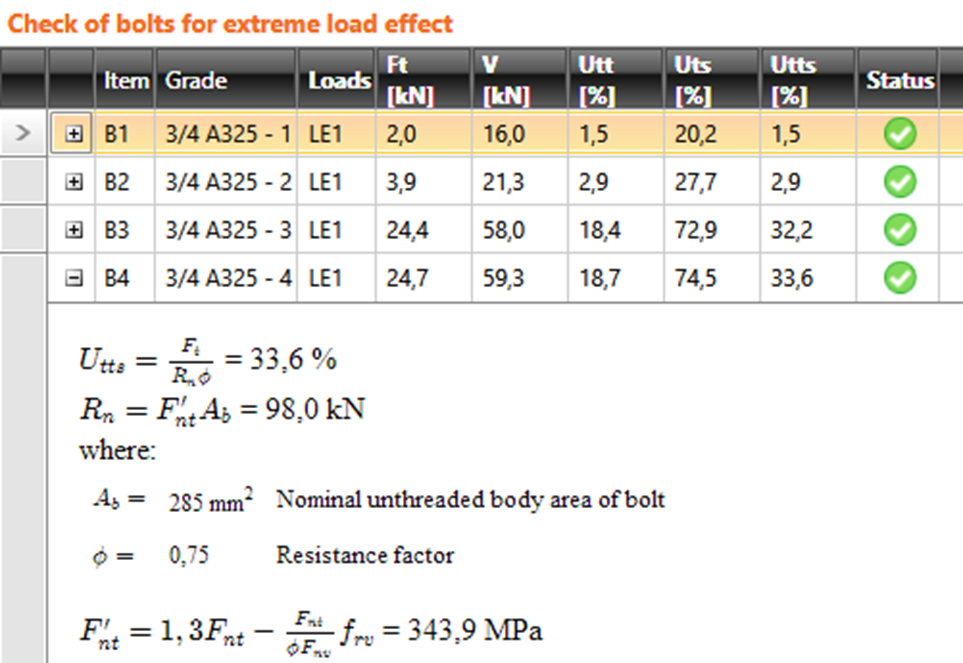### Choose language# How do checks in IDEA StatiCa Connection align with the code

$$The check of joint in a standard component-based method and in the CBFEM method used in IDEA StatiCa Connection is based on the check of all parts of joint – the components. Components can be bolts, anchors, welds, cross-sections and plates, concrete at the footing. The check itself consists of two steps: Forces in each component of the joint are calculated Each component is checked due to the code equations Add 1 – Calculation of forcesThe Component-based method makes the behaviour of each component simpler and it’s not general method. IDEA StatiCa Connection uses the most accurate model by using the finite element method. Forces are calculated without simplifying presumptions and the other effects like the interaction of the components, praying etc. are calculated too. Nothing is neglected.Add 2 – ChecksThe checks are performed for the calculated forces by the same equations as in the code in all methods. Equations used for the bolts, anchors, welds and concrete in the footing are presented in the application and can be reviewed.The plates are in IDEA StatiCa Connection calculated even more accurate. Therefore, the application checks only the yield of the strength and limit of plastic strain.$$